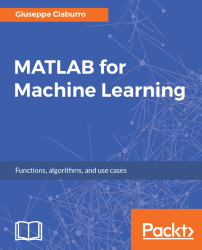•#### MATLAB for Machine Learning#### Overview of this book

MATLAB is the language of choice for many researchers and mathematics experts for machine learning. This book will help you build a foundation in machine learning using MATLAB for beginners. You’ll start by getting your system ready with t he MATLAB environment for machine learning and you’ll see how to easily interact with the Matlab workspace. We’ll then move on to data cleansing, mining and analyzing various data types in machine learning and you’ll see how to display data values on a plot. Next, you’ll get to know about the different types of regression techniques and how to apply them to your data using the MATLAB functions. You’ll understand the basic concepts of neural networks and perform data fitting, pattern recognition, and clustering analysis. Finally, you’ll explore feature selection and extraction techniques for dimensionality reduction for performance improvement. At the end of the book, you will learn to put it all together into real-world cases covering major machine learning algorithms and be comfortable in performing machine learning with MATLAB.
Title PageCreditsForewordwww.PacktPub.comCustomer FeedbackPrefaceFree Chapter
Getting Started with MATLAB Machine LearningImporting and Organizing Data in MATLABFrom Data to Knowledge DiscoveryFinding Relationships between Variables - Regression TechniquesPattern Recognition through Classification AlgorithmsIdentifying Groups of Data Using Clustering MethodsSimulation of Human Thinking - Artificial Neural NetworksImproving the Performance of the Machine Learning Model - Dimensionality ReductionMachine Learning in Practice## Summary

In this chapter, we learned how to select a feature that best represents a set of data. We gained an understanding the basic concept of dimensionality reduction. We saw how to perform a feature extraction procedure for dimensionality reduction when transformation of variables is possible. We also explored stepwise regression and PCA.

We learned how to use the `stepwiselm()` function to create a linear model and automatically add/remove variables from the model. We also saw how to create a small model starting from a constant model, and how to create a large model starting from a model containing many terms. We reviewed the methods to remove missing values from a dataset.

Subsequently, we covered the techniques for extracting features. In particular, we analyzed PCA. PCA is a quantitatively rigorous method for achieving this simplification. The method generates a new set of variables, called principal components. Each principal component is a linear combination of the original variables...